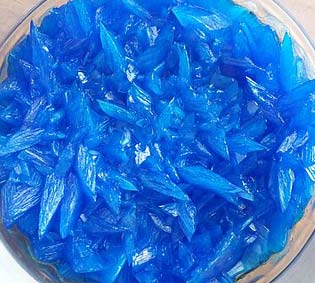Chemistry Edexcel GCSE:
Calculations 2a

For Physics / Triple science only

Grab a calculator and a copy of the periodic table

(This test has only 10 questions, but may take 15 - 20 mins).

Q1-2:
A student produced a sample of copper(II)sulfate crystals by reacting excess copper(II)oxide with dilute sulfuric acid. The excess copper(II)oxide was removed by filtration and the crystals formed by crystallization of the copper(II)sulfate solution.
She calculated that she should obtain 18.6g of dry crystals but she only actually obtained 12.4g.1. What was her percentage yield?
• A.   1.5%
• B.   150%
• C.   0.67%
• D.   67%
2. Which of the following is NOT a reason for the actual yield of copper(II)sulfate crystals being less than the theoretical yield?
• A.   The reaction between copper(II)oxide and sulfuric acid was incomplete
• B.   Some copper(II)sulfate solution was lost during filtration
• C.   Some copper(II)sulfate solution did not crystallise
• D.   The copper(II)sulfate crystals were still damp
3. Which solution contains the greatest number of moles?
• A.   20 cm3 of 2.0 mol dm-3 hydrochloric acid
• B.   40 cm3 of 1.0 mol dm-3 hydrochloric acid
• C.   60 cm3 of 1.0 mol dm-3 sodium hydroxide
• D.   80 cm3 of 0.5 mol dm-3 sodium hydroxide
4. 1.0dm3 of ethanoic acid solution, CH3COOH, contains 6.0 g of ethanoic acid

[Mr value of CH3COOH = 60].
The concentration of the ethanoic acid solution, in mol dm-3, is..

• A.   0.1
• B.   0.6
• C.   1.0
• D.   10
5. Sodium hydroxide solution can be neutralized using dilute ethanoic acid. The equation for the reaction is:

NaOH(aq) + CH3COOH(aq)  CH3COONa(aq) + H2O(l)

Calculate the volume of 0.200 mol dm-3 ethanoic acid needed to exactly neutralize 0.0025 moles of sodium hydroxide solution.

• A.   0.0125 cm3
• B.   1.25 cm3
• C.   12.5 cm3
• D.   12.5 dm3

6. An equation for the reaction between carbon and nickel oxide is:

NiO  + C → Ni + CO

Calculate the percentage atom economy for this reaction to produce nickel.
[Ar of C = 12; Ar of Ni = 59; Mr of NiO = 75; Mr of CO = 28]

• A.   33.0%
• B.   51.3%
• C.   67.8%
• D.   78.7%
7. A student calculated she should produce 20.0g of aspirin in a reaction.

If the percentage yield for this reaction was only 72% what mass of aspirin did the student actually produce?

• A.   7.2g
• B.   14.4g
• C.   27.8g
• D.   1440g
8. 0.30 moles of barium chloride solution was added to 0.20 moles of magnesium sulfate solution in the following precipitation reaction:

BaCl2(aq) + MgSO4(aq)  BaSO4(s) + MgCl2(aq)

How many moles of barium sulfate, BaSO4, would be produced?

• A.   0.20
• B.   0.25
• C.   0.30
• D.   0.50
9. When choosing a particular reaction pathway to produce a product a manufacturer would ideally look for a reaction which had a ..
• A.   low percentage atom economy and a high percentage yield
• B.   high percentage atom economy and a low reaction rate
• C.   low percentage atom economy and a high reaction rate
• D.   high percentage atom economy and a high percentage yield
10. 100cm3 of propane gas was burned in oxygen in the following reaction:

C3H8(g) + 5O2(g) 3CO2(g) + 4H2O(l)

What volume of oxygen gas was used and what volume of carbon dioxide was formed?

 Volume of oxygen used (cm3) Volume of carbon dioxide formed (cm3) A 500 100 B 500 300 C 100 100 D 100 300